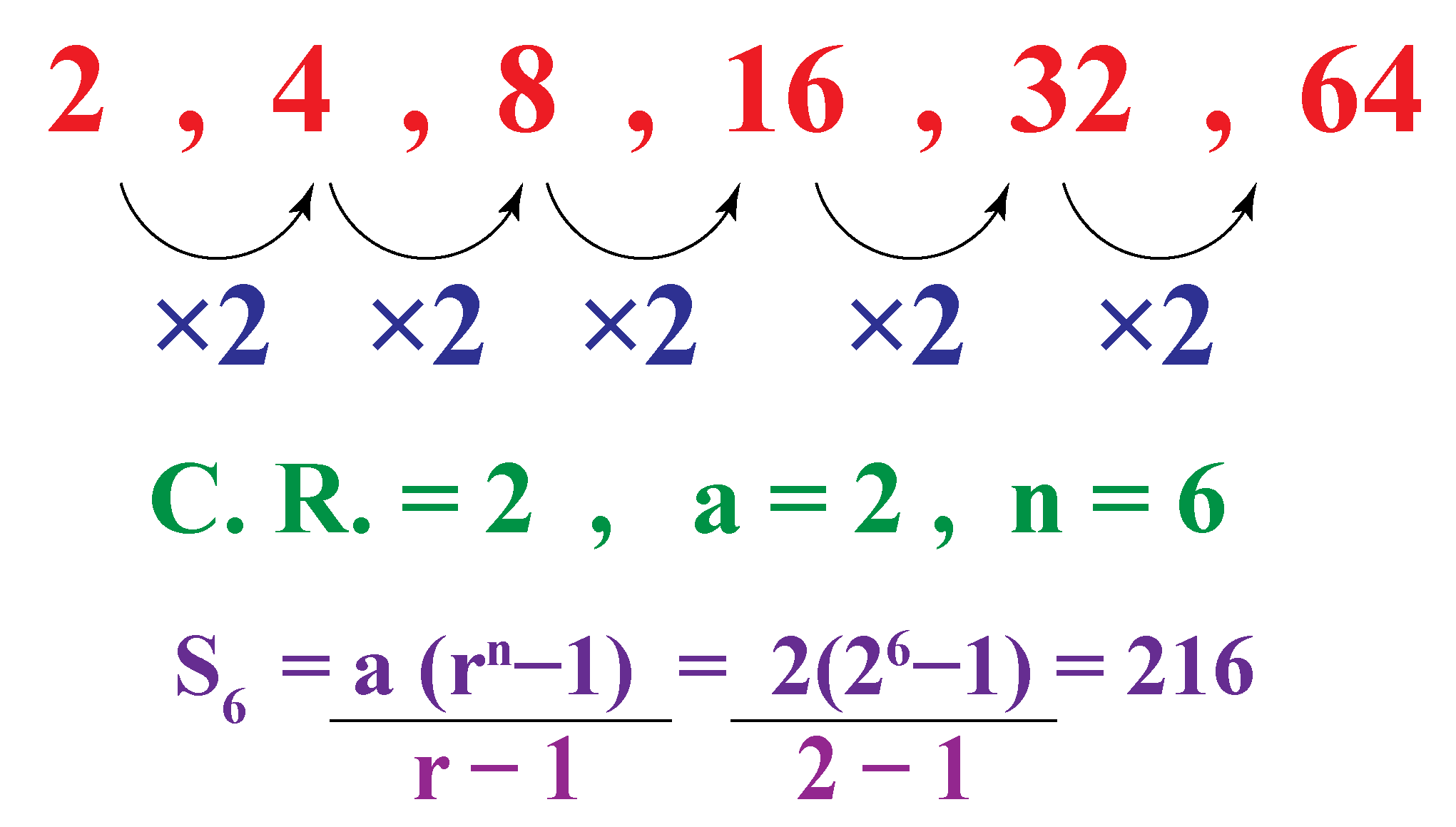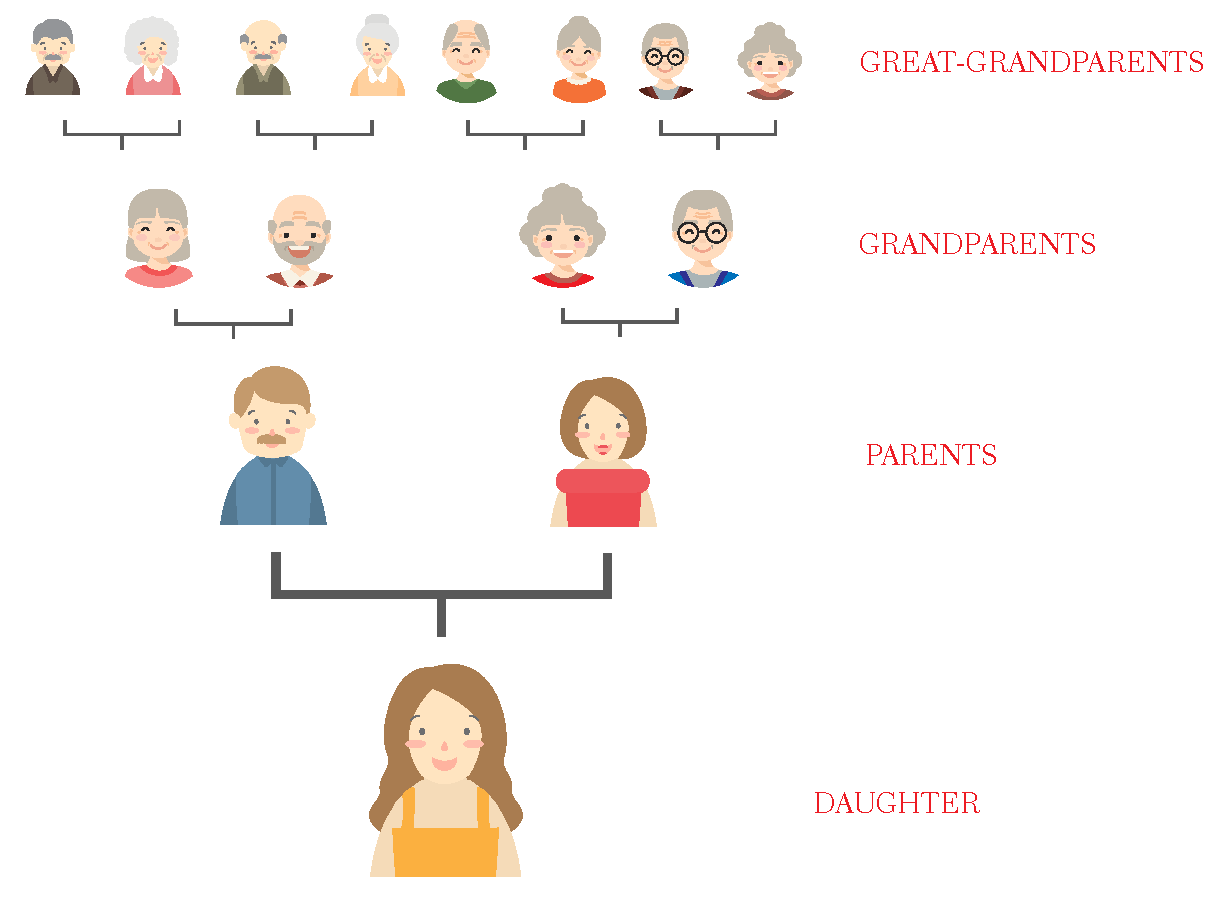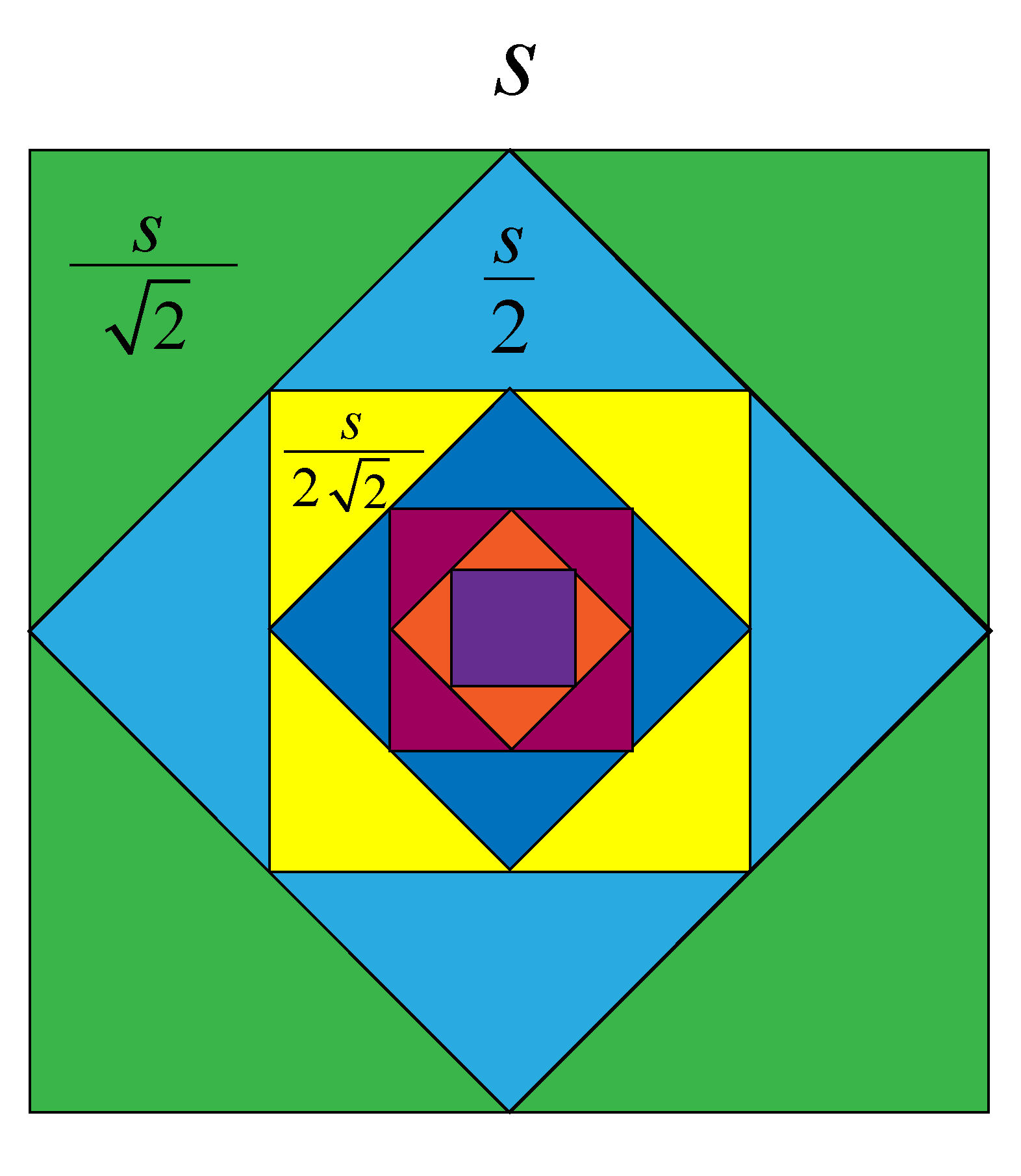# Sum of a GP

Sum of a GP

In this mini-lesson, we target finding the sum of a GP. Let us learn to find the sum of n terms of a GP, sum of infinite GP, the sum of GP formula, the sum of terms in GP, the sum of finite GP, the sum of infinite terms in GP, the sum of geometric progression

Clara saves a few dollars every week in a particular fashion. In week 1 she deposits $2. In week 2 -$4, in week 3 - $8, in week 4 -$16, and so on. How much will she have at the end of 6 weeks in her piggy bank?A series of numbers obtained by multiplying or dividing each preceding term, such that there is a common ratio between the terms (that is not equal to 0) is the geometric progression and the sum of all these terms formed so is the sum of the geometric progression.

Here, the amount accumulated every week has a constant ratio of $2 and the initial value is$2.

The amount deposited every week would be $2,$4, $8,$16, $32,$64.

So, at the end of 6 weeks she will have $2+$4 + $8+$16+ $32 +$64 + \$126 accumulated in her piggy bank.

We can find the same using the formula of the sum of GP of "n" terms. Let's learn to calculate it now.

## Lesson Plan

 1 What Is the Sum of a Geometric Progression? 2 Important Notes on Sum of a GP 3 Solved Examples on Sum of a GP 4 Challenging Questions on Sum of a GP 5 Interactive Questions on Sum of a GP

## What Is the Sum of Geometric Progression?

Let's discuss how to sum an arbitrary GP. Consider the sum of the first n terms of a GP with first term $$a$$ and common ratio $$r$$.

Sum of the geometric progression is:

$S = a + ar + a{r^2} + ... + a{r^{n - 1}}$

Multiply both sides by r, and write the terms with the same power of r below each other, as shown below:

\begin{align}S & = a + ar + a{r^2} + ... + ar^{n - 1}\\\\rS &= ar + ar^2 + ... + ar^{n - 1}+ a{r^n}\end{align}

Now, subtract the first relation from the second relation:

$(r - 1)S = a{r^n} - a$

Thus the sum of GP formula if r>1 is given by:

 $$S_n = \dfrac{a(r^n-1)}{r - 1}, r\neq 1$$

Thus the sum of GP formula if r<1 is given by:

 $$S_n = \dfrac{a(1- r^n)}{1 - r}, r\neq 1$$

If $$r = 1$$,

 $$S_n = na$$

Considering the above said piggy bank problem, the sum of the GP is found in the following way.### Sum of a GP

Note the ball being dropped from a height above in the animation below.

Adjust its height(a) and time(r) in the sliders shown at the top.

The ball loses its energy and the sequence of maximum heights is approximately geometric.

Find the total time taken by it from its bounce time to the time it comes to rest.

### Examples

1) Consider the GP of this ancestral tree.

The terms are: $1, 2, 4, 8,16,...$

We have $$a = 1$$, and $$r = 2$$. Now, let us find the sum of the first 12 generations of this GP.

\begin{align}{S_{12}} &= \dfrac{ (1) (2^{12} - 1)} {2 - 1}\\\\ &= 2^{12} - 1\\\\ &= 4095 \text{ people in 12 generations}\end{align}2) Consider another GP:

$\dfrac{1}{2}, \dfrac{1}{6},\dfrac{1}{18},...$

We have:

$a = \dfrac{1}{2},\,\,\,\,r = \dfrac{1}{3}$

Now, the sum of the first 6 terms in this GP will be:

\begin{align}&{S_6} = {\dfrac{1}{2}} \dfrac{(1-(\dfrac{1}{3})^6)}{1- \dfrac{1}{3}} \\\\&= \dfrac{1}{2} \dfrac{(1- \dfrac{1}{729})} {(\dfrac{2}{3})}\\\\&=\dfrac{182}{243}\\\\ &= 0.76\end{align}

## How to Calculate the Sum of n Terms of a GP?

### Sum of Finite GP

Identify the geometric progression and determine the common ratio "r" and the first term"a".

Find the number of terms "n" for which the sum has to be performed.

### Sum of Infinite GP

To find the sum of an infinite Geometric progression, we have the first term and the constant ratio between the terms. We wouldn't know the last term. Then, $$s_n = \dfrac{a_1}{1-r}$$

• If $$\mid r \mid < 1$$, then the series converges and the series has a sum.

For example, A square is drawn by joining the midpoints of the sides of the original square. A third square is drawn inside the second square in the same way and this process is continued indefinitely. If a side of the first square is "s" units, determine the sum of areas of all the squares so formed?The GP thus formed is: $s^2, \dfrac{s^2}{2},\dfrac{s^2}{4}, \dfrac{s^2}{8}...........$

Taking $$s^2$$ out, we get,

$s^2(1+ \dfrac{1}{2} + \dfrac{1}{4} + \dfrac{1}{8}...)$

$r = \dfrac{\dfrac{1}{2}}{1} = \dfrac{1}{2}$

Here, $\mid r \mid = \dfrac{1}{2} < 1$

\begin{align}\text{sum of the GP}&= s^2(S_\infty)\\\\ &= s^2(\dfrac{1}{1-\dfrac{1}{2}})\\\\ &= 2 s^2\end{align}

 $$s_n = \dfrac{a_1}{1-r}$$

### Sum of Infinite GP Calculator

Find the simulation below. Enter the first term and the common ratio and check how the sum of the infinte GP getting converged to a smaller value.

• If $$\mid r \mid > 1$$,then the series does not converge and it has no sum. It is divergent.

For example: Consider the infinite series of the sum of reciprocal of primes,

$-\dfrac{5}{10}+ \dfrac{15}{10} -\dfrac{45}{10} + \dfrac{135}{10}+.......$

We find the initial term is $a= \dfrac{5}{10}$ and

The common ratio is $r =\dfrac{\dfrac{15}{10}}{-\dfrac{5}{10}} = -3$

Here $$\mid r \mid > 1$$  that is $$3 > 1$$ and the magnitudes of the terms keep getting larger.

The sum of this GP tends to diverge and not converge to a value.

## Solved Examples

 Example 1

In a GP, the sum of the first three terms is 16, and the sum of the next three terms is 128. Find the sum of the first n terms of the GP.

Solution

Let a and r be the first term and the common ratio of GP. We have:

$\begin{array}{l}\left\{ \begin{array}{l}a + ar + a{r^2} = 16\\a{r^3} + a{r^4} + a{r^5} = 128\end{array} \right.\\ \Rightarrow \,\,\,\left\{ \begin{array}{l}a\left( {1 + r + {r^2}} \right) = 16\\a{r^3}\left( {1 + r + {r^2}} \right) = 128\end{array} \right.\end{array}$

Dividing these two relations we get,

\begin{align}\dfrac{ar^3 (1 + r + r^2)}{a(1 + r+ r^2)} = \dfrac{128}{16}\\\\\Rightarrow r^3 = 8 \\\\\Rightarrow r &= 2\end{align}

Substituting this in any of the two relations gives $$a = \frac{{16}}{7}$$. The sum of the first n terms of the GP will be:

${S_n} = \frac{{\left( {\frac{{16}}{7}} \right)\left( {{2^n} - 1} \right)}}{{2 - 1}} = \frac{{16\left( {{2^n} - 1} \right)}}{7}$

 $$\therefore$$ The sum of n terms of this GP $$=\dfrac{16(2^n-1)}{7}$$
 Example 2

Sara shared a message with 5 unique people at 1 am. At 2 am, each of her friends shared it with 5 unique people. Then at 3 pm each of their friends shared with 5 unique people. In this sequence, how many unique people would have received the message by 8 am?Solution

Clearly, we find that this is a geometric progression as the first term is 5

Common ratio $$= \dfrac{25}{5} = \dfrac{125}{25} = 5$$

\begin{align}S_8 &= \dfrac{5(5^8 - 1)}{5 - 1} \\\\&=\dfrac{5(390624)}{4}\\\\ &= 5\times 97656\\\\&= 488,280\text{ people}\end{align}

 $$\therefore$$ 488,820 people would have received the message by 8 am.
 Example 3

The distance travelled by a ball dropped from a height (in inches) are $$\dfrac{128}{9}, \dfrac{32}{3}$$, 8, 6... What could be the distance traveled by the ball before coming to rest?Solution

The distance travelled by the ball $$= \dfrac{128}{9}, \dfrac{32}{3}, 8, 6...$$

Here the initial term $$a = \dfrac{128}{9}$$ and the common ratio is:

\begin{align}r&=\dfrac{(\dfrac{32}{3})}{(\dfrac{128}{9})}\\\\&=\dfrac{32}{3}\times\dfrac{9}{128}\\\\&=\dfrac{3}{4}\end{align}

The total distance traveled by the ball will be the sum of this infinite GP.

Sum of infinite GP: \begin{align} &= \dfrac{a}{1-r}\\\\&= \dfrac{\dfrac{128}{9}}{1 - \dfrac{3}{4}}\\\\&=\dfrac{128}{9}\times 4\\\\&= \dfrac{512}{9}\text{ in}\\\\ &= 56.88 \text{ in}\end{align}

 $$\therefore$$ Total distance = 56.88 inChallenging Questions
• If the sum of 3 real numbers in a GP is 26 and the sum of their squares is 364, find the largest number.
• Find the sum of the infinite GP 0.3+ 0.33+ 0.333+.... [hint: $$0.3 = \dfrac{3}{10}$$]

## Interactive Questions

Here are a few activities for you to practice. Select/Type your answer and click the "Check Answer" button to see the result.

## Let's Summarize

The mini-lesson targeted the fascinating concept of the sum of a GP. The math journey around the sum of a GP started with what a student already knew and went on to creatively crafting a fresh concept in the young minds. Done in a way that not only it is relatable and easy to grasp, but also will stay with them forever.

At Cuemath, our team of math experts is dedicated to making learning fun for our favorite readers, the students!

Through an interactive and engaging learning-teaching-learning approach, the teachers explore all angles of a topic.

Be it worksheets, online classes, doubt sessions, or any other form of relation, it’s the logical thinking and smart learning approach that we, at Cuemath, believe in.

## 1. What is the full form of GP?

The full form of GP is Geometric Progression.

## 2. What is a GP in maths?

A sequence in Math obtained where the consecutive terms are in the constant ratio is called the geometric progression.

## 3. How do you identify a geometric sequence?

In a geometric sequence, any two terms are in a constant ratio.

## 4. What is the rule for a geometric sequence?

The ratio between the terms is constant. i.e., except for the first term, the other terms are found by multiplying the previous term by a constant.

## 5.What is the formula for the sum of GP?

The formula for sum of GP is $S_n = \dfrac{a(r^n-1)}{r - 1}$

## 6. What is the sum of infinite GP?

$S_\infty = \dfrac{a}{1-r}$

## 7. How do you find the sum of the first 10 terms of a geometric progression?

\begin{align}S_n &= \dfrac{a(r^n-1)}{r - 1}\\S_{10} &= \dfrac{a (r^9- 1)}{r - 1}\end{align}

Example, the sum of first 10 terms in the GP, 3, 6, 12,..... is given as

\begin{align}S_{10} &= \dfrac{3(r^{10}-1)}{2 - 1}\\S_{10} &= \dfrac{3 (2^{10}- 1)}{2 - 1}\\&=3(1024-1)\\&= 3\times 1023 \\&=3069\end{align}

## 8. What is r in GP?

The common ratio between the consecutive terms is r in GP.

## 9. How do you find the sum of infinite terms?

$S_\infty = \dfrac{a}{1-r}, -1 < r <1$

## 10. What is the formula for the last term of GP?

$\text{The last term of GP} = a r^{n-1}$

More Important Topics
Numbers
Algebra
Geometry
Measurement
Money
Data
Trigonometry
Calculus
More Important Topics
Numbers
Algebra
Geometry
Measurement
Money
Data
Trigonometry
Calculus